﻿ 基于形态学的滤波消除泥浆脉冲信号基线漂移 Removing Baseline Drifting in Mud Pulse Signals Based on Morphological Filtering

Journal of Oil and Gas Technology
Vol. 41  No. 04 ( 2019 ), Article ID: 32035 , 6 pages
10.12677/JOGT.2019.414049

Removing Baseline Drifting in Mud Pulse Signals Based on Morphological Filtering

Chaoyang Xu, Xianghui Ren, Qiang Xue, Qianhong Cao

Directional Drilling Service Company, CNPC Bohai Drilling Engineering Company Limited, Tianjin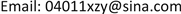Received: Mar. 20th, 2019; accepted: Apr. 18th, 2019; published: Aug. 15th, 2019ABSTRACT

A morphological filter for removing mud pulse baseline drifting was established. The baseline drifting of simulated PPM encoding signal of mud pulse was processed by least-square polynomial fitting, median filtering and morphological filtering methods, respectively. The results were quantitatively analyzed by using signal to noise ratio (SNR), root mean square error (Erms) and normalized correlation coefficient (Cncc). The analysis result shows that the morphological filtering method proposed is the best for removing the baseline drift of mud pulse signal.

Keywords:MWD, Mud Pulse Signal, Baseline Drift, Morphological Filtering1. 引言

2. 泥浆脉冲信号噪声干扰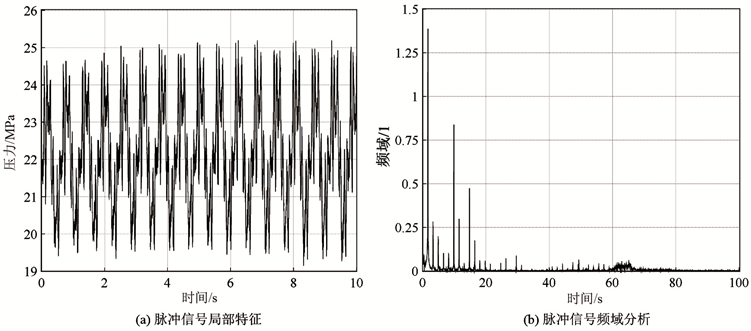Figure 1. The field acquisition of mud pressure pulse signal and frequency domain analysis of pulse signal

3. 形态学滤波

3.1. 形态学运算

f(n)关于g(m)腐蚀的关系式如下：

$\left(f\Theta g\right)\left(n\right)=\mathrm{min}\left\{f\left(n+m\right)-g\left(m\right)\right\}$$n=0,\text{1},\cdots ,N-M$ (1)

f(n)关于g(m)膨胀的关系式如下：

$\left(f\oplus g\right)\left(n\right)=\mathrm{max}\left\{f\left(n-m\right)+g\left(m\right)\right\}$$n=0,\text{1},\cdots ,N+M-\text{2}$ (2)

$\left(f\circ g\right)\left(n\right)=\left(f\Theta g\oplus g\right)\left(n\right)$ (3)

$\left(f•g\right)\left(n\right)=\left(f\oplus g\Theta g\right)\left(n\right)$ (4)

3.2. 形态学滤波器设计

${f}_{1}=\frac{1}{2}\left({f}_{0}\circ {g}_{1}•{g}_{1}+{f}_{0}•{g}_{1}\circ {g}_{1}\right)$ (5)

${f}_{2}=\frac{1}{2}\left({f}_{1}\circ {g}_{2}•{g}_{2}+{f}_{1}•{g}_{2}\circ {g}_{2}\right)$ (5)

${f}_{3}={f}_{0}-{f}_{2}$ (6)

4. 试验分析

4.1. 试验样本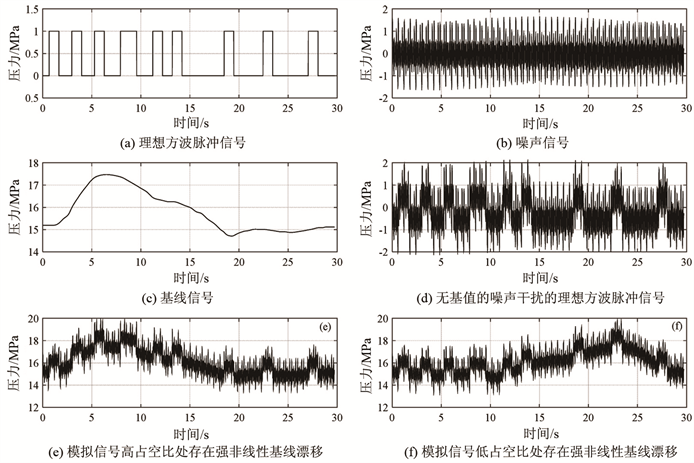Figure 2. The signals of test samples

4.2. 结果分析

4.2.1. 定性分析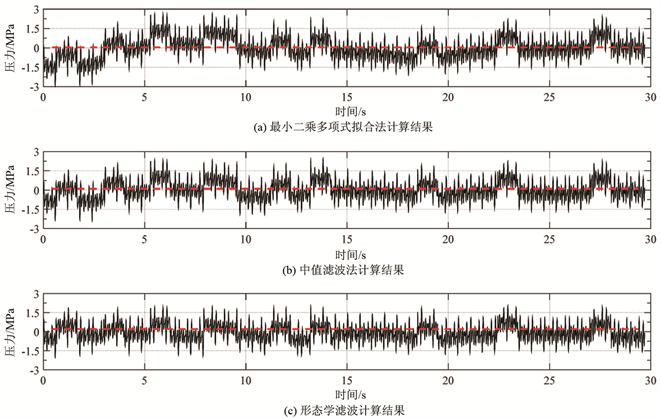Figure 3. The modified results of strong nonlinear baseline drift at high duty cycle of analog signals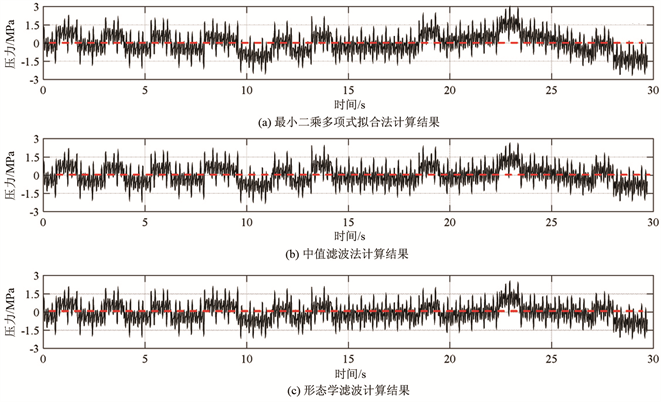Figure 4. The modified results of strong nonlinear baseline drift at low duty cycle of analog signals

4.2.2. 定量分析Table 1. The comparison of modified results of strong nonlinear baseline drift at high duty cycle of analog signalsTable 2. The evaluation of modified results of strong nonlinear baseline drift at low duty cycle of analog signals

5. 结论

 梁耀. 随钻泥浆脉冲信号的处理[D]: [硕士学位论文]. 西安: 西安石油大学, 2013: 21-37.

 邱彬, 周静, 高建邦, 等. 随钻测量信号中泵冲噪声对消[J]. 石油机械, 2014, 42(12): 22-27.

 陈伟, 李晓林, 江佩佩. 基于2D-DCT变换的MWD遥传信号泵冲干扰消除方法[J]. 测控技术, 2016, 35(10): 19-22.

 廖琪梅, 李安宗, 屈景辉, 等. 随钻测井钻井液脉冲信号基线漂移的矫正[J]. 石油钻采工艺, 2008, 30(4): 41-43.

 郭晓鑫, 刘立, 冯志鹏. 数学形态学在信号处理中的应用综述[J]. 仪器仪表学报, 2009, 30(10): 187-191.

 刘艳丽, 赵为松, 李海坤, 等. 基于形态滤波的脉搏波信号基线漂移消除方法研究[J]. 合肥工业大学学报(自然科学版), 2011, 34(4): 525-528.

 庞宇, 邓璐, 林金朝, 等. 基于形态滤波的心电信号去除基线漂移方法[J]. 物理学报, 2014, 63(9): 1-5.

[编辑] 帅群